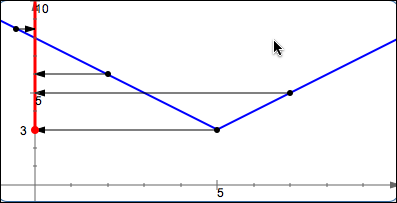﻿ Finding the Domain and Range of a Function

# FINDING THE DOMAIN AND RANGE OF A FUNCTION

• PRACTICE (online exercises and printable worksheets)

Read Domain and Range of a Function from the Algebra I curriculum for a basic introduction to domain and range.

## Finding the domain of a function from a formula

The following situations are not allowed, so you must exclude value(s) that cause:
• division by zero
• even roots of negative numbers
• zero raised to the zero power

EXAMPLE:
Find the domain of $\displaystyle\,g(x) = \frac{\sqrt{x-3}}{x-5}\,$.

 SOLUTION: $x-3\not\lt 0\ \text{ and }\ x-5\ne 0$ $x-3\ge 0 \ \text{ and }\ x-5\ne 0$ $x\ge 3\ \text{ and }\ x\ne 5$ $\text{dom}(g) = [3,5) \cup (5,\infty)$## Finding the domain of a function from a graph

‘Collapse’ each point into its $x$-value.

## Finding the range of a function

The range of a function is its output set.
It's easiest to find the range when you have the graph of the function!
‘Collapse’ each point into its $y$-value.

EXAMPLE:

Find the range of $\,f(x) = |x-5| + 3\,$.

 SOLUTION: Graph $\,f\,$ by starting with $\,y = |x|\,$, shifting it right $\,5\,$ and up $\,3\,$. $\text{ran}(f) = [3,\infty)$Master the ideas from this section